Recently I’ve been working on a project related to Named Entity Recognition (NER). At the very beginning, I was trying to find a well-explained document to get myself started, but couldn’t do so (instead I found redundant pieces here and there on the Internet). My requirement is simple. It should include

• what is NER

• how to formulate it

• what are the traditional and start-of-the-art models

• what are the off-the-shelf options

• how to build a customized NER model

So this post will try to provide a complete set of explanation on these questions.

## 1. What is NER

Simply put, Named Entity Recognition is a technology that identifies certain entities from a sentence/paragraph/document. Like the sentence below, NER tries to tag each word with a label; Steve Jobs and Steve Wozniak to be PER (persion entity), Apple to be ORG (organization entity) and the rest O (not an entity).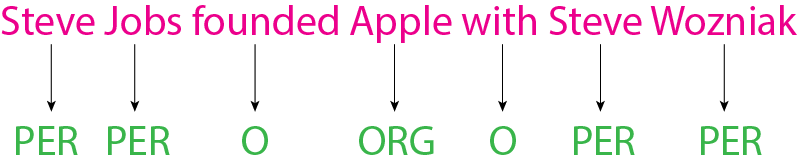This is useful in lots of cases. For instance, Apple Mail identifies TIME entity in emails and makes pulling events from email to calendar much easier than before; Google Search is able to find Headquarters entity in a relevent document to answer query questions.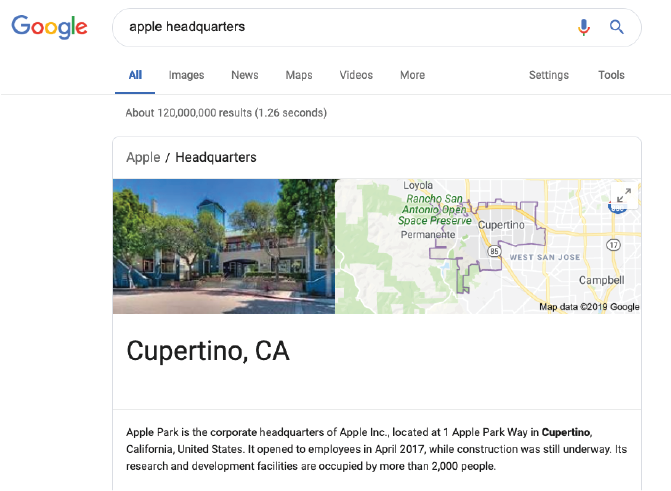So how does it actually work?

## 2. Mathematical Formulation

Let’s step back and see what NER is doing essentially. For a given sentence $x_1 ... x_n$, NER decides to tag each word $x_i$ with an entity label $y_i$.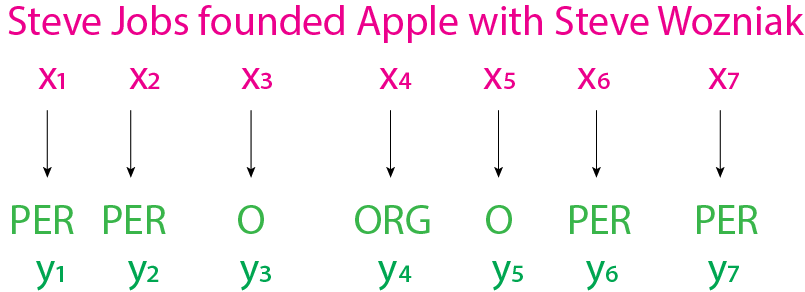One obvious challenge here is one word should be tagged differently depending on its context. E.g., Apple in the example above is an organization entity, but in other contexts might be a fruit entity.

So how can we tag wisely? Basically we need two things.

• a score function $s(y_1...y_n, x_1...x_n)$, which splits out a score measuring how fit a tagging sequence $y_1 ... y_n$ is to a given sentence $x_1 ... x_n$. A well-designed score function should assign a higher score to a tagging sequence that fits better.

• a solver, which is able to pick the highest-scoring tagging sequence among overwhelmingly large number of possible candidates. Just take a sentence with $7$ words as an example, we are talking about $10^7$ tagging candidates if there’s 10 unique entities to choose from. A good solver should be able to efficiently get to the best tagging sequence.

### 2.1 Score Function

Researchers in the field like to use probability model to build score function: a better-fitting sequence can be given a higher probability. Often times we choose conditional probability (one can use joint probablity as well), like below

If we make a simplication $p(y_i |y_1...y_{i-1}, x_1...x_n) \approx p(y_i | y_{i-1}, x_1...x_n)$, then

Now the question becomes how do we model

#### 2.1.1 Model $p(y_i | y_{i-1}, x_1...x_n)$

Following the above example, we are basically asking how likely Jobs is PER / ORG / MISC / O, if Steve is PER?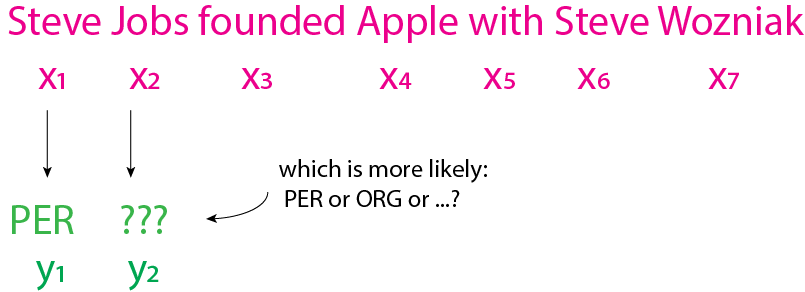A natural thought would be to create a second score function (let’s call it local score function since it looks only at $y_i, y_{i-1}$) with well-defined local features $\vec{f}(y_{i-1}, y_i, x_1...x_n)$:

and define probability based on the local score function:

$\vec{f} \in R^m$, is an m-dimension vector, meaning there’s m predefined feature functions $f_1...f_m$. Some feature examples could be:

At this point, once we have defined $\vec{f}$ and picked the weights $\theta$ for the features, we can readily calculate $p(y_i | y_{i-1}, x_1...x_n)$ and tagging score from

#### 2.1.2 Three remaining questions

Let’s summarize here. With this framework set up, there’s only 3 questions remaining:

• how to design feature functions $\vec{f}(y_{i-1}, y_i, x_1...x_n)$?

Traditionally it’s kinda art and requires expert knowledge in the problem domain. But recent deep learning approaches such as LSTM, BiLSTM aim to utilize large neural networks and automatically figure out suitable featurization from data.

• how to estimate weights $\vec{\theta}$?

$\vec{\theta}$ can be obtained by training on data. One intuitive and popularly used method is Maximum Likelihood Estimation.

• assuming we already know $\vec{f}, \vec{\theta}$, how to get the best fitting tag sequence $y_1^{*}...y_n^{*}$ for a sentence $x_1...x_n$?

This will be the central topic in the next section - Solver section.

### 2.2 Solver

Now let’s assume we’ve determined $\vec{f}, \vec{\theta}$ from expert knowledge and/or data, so our score function $s(y_1...y_n, x_1...x_n)$ is finalized.

The job of the solver here is use the score function to efficiently get the best fitting tag sequence $y_1^{*}...y_n^{*}$ for a given sentence $x_1...x_n$. It’s an optimization problem:

We can definitely solve it by brutal force - it’s just that there’s $5^7$ possible $y_1...y_n$ sequences in the example below, meaning we have to evaluate $5^7$ times of our score function before getting the optimal sequence.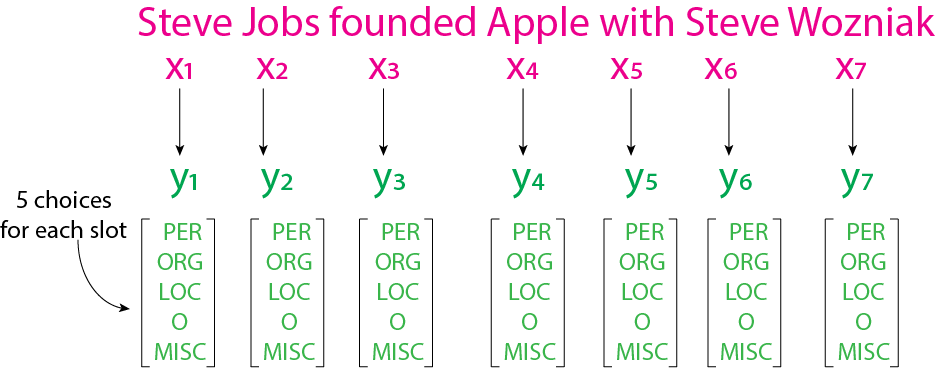The computation scales badly with the length of sentence.

The computation complexity is exponential - $O(5^N)$ where $N$ is the length of sentence.

It turns out the score function exhibits a special mathematic structure that enables us to solve the optimization problem in linear time $O(N)$. Let’s talk about that.

#### 2.2.1 Viterbi Algorithm

We notice our score function is a product of successive conditional probabilities $p(y_i |y_{i-1}, x_1...x_n)$ as below:

You’ll see this is a very good property that allows us to optimize step by step via dynamic programming (in this case we call Viterbi Algorithm). So, bear with me for a bit…

Because of this nice and clean form, we can easily define a partial score function $s_{partial}$ - score of the first $k$ tags $y_1...y_k$ for the same given sentence $x_1...x_n$.

When $k=n$, the partial score function becomes the original score function.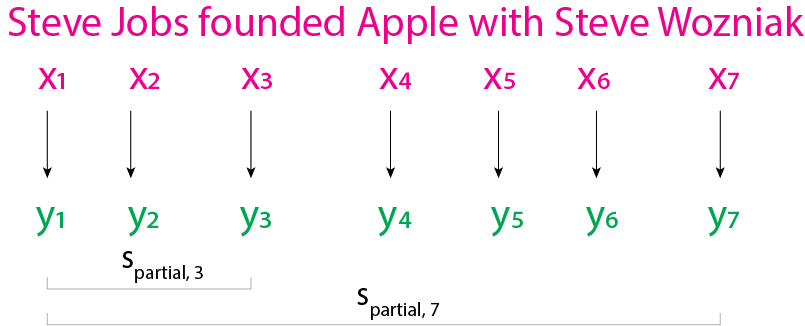We can also easily find the recursive form of the partial score function:

Now, we only need answer three questions to explain the algorithm.

• How would you find highest partial score where $k=1$, namely $s_{partial, 1}(y_1^{*}, x_1...x_n)$?

This is easy because it’s just a single-variable optimization and $y_1$ can only have 5 choices.

We can just evaluate $s_{partial, 1}(y_1, x_1...x_n)$ for 5 times and pick the highest one. To help answer the following questions, we’ll save the 5 evaluated numbers. Here we carried out $5$ evalutions to get $s_{partial, 1}(y_1^{*}, x_1...x_n)$.

• How would you find highest partial score where $k=2$, namely $s_{partial, 2}(y_1^{*}, y_2^{*}, x_1...x_n)$?

It’s just bi-variable optimization. We can first keep $y_2$ fixed and optimize over $y_1$ dimension.

We can calculate the best $s_{partial, 2}(y_1^{*},y_2=\text{PER}, x_1...x_n)$ by evaluating the following 5 products and pick the best one.

Note we can reuse $s_{partial, 1}(y_1, x_1...x_n), y_1 \in \{\text{PER, ORG, LOC, MISC, O}\}$ saved from previous question.

In this manner, we have all of $s_{partial, 2}(y_1^{*},y_2, x_1...x_n), y_2 \in \{\text{PER, ORG, LOC, MISC, O}\}$ ready and the highest of the five would be the answer. Here we carried out $5*5=25$ evalutions to get $s_{partial, 2}(y_1^{*}, y_2^{*}, x_1...x_n)$.

• How about $k=3$ and beyond?

At this moment, you might have noticed that we could get $s_{partial, 3}(y_1^{*}, y_2^{*}, y_3^{*}, x_1...x_n)$ by evaluating $s_{partial, 3}(y_1^{*},y_2^{*}, y_3, x_1...x_n), y_3 \in \{\text{PER, ORG, LOC, MISC, O}\}$ and picking the highest of the five.

Each $s_{partial, 3}(y_1^{*},y_2^{*}, y_3=u, x_1...x_n)$ can be easily calculated via

Note we can reuse $s_{partial, 2}(y_1^{*}, y_2, x_1...x_n), y_2 \in \{\text{PER, ORG, LOC, MISC, O}\}$ saved from previous question.

Similarly, it takes another 25 evalutions. Basically we can keep rolling to all the way $k=n$ with each step forward we carry out another 25 evaluations. So the total compuation complexity is around $25N$, namely $O(N)$.

This post turns out to be longer than I thought, I was only able to answer the first questions raised in the beginning. And this seemingly natural approach is actually the so-called maximum entropy Markov model (MEMM) approach. There’s many more other ways to solve NER problem, which I’ll talk about in next posts together with the answers to the remaining three questions. See you soon.